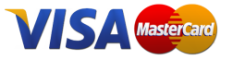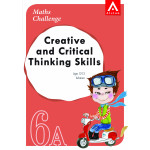#### InformationView larger

# Maths Challenge - Creative and Critical Thinking Skills 6A (Advance)

Maths Challenge uses the power of Creative Mathematics to solve mathematical problems, which are much like puzzles that we can imagine and visualise in everyday objects such as bathroom room tiles and playing blocks. Fitting the pieces of these puzzles together is a skill that can be applied to solving problems in mathematics.

R130.00 VAT incl.You need a strong spatial sense to build up your confidence in puzzle solving. Maths Challenge is designed to help you to sharpen your skills in this area. Using this skill, you can form creative and logical equations to represent mathematical problems, and work towards a solution from there. This is how Creative Mathematics works.

Maths Challenge is a series of 14 books that are developed to harness this method of creative problem-solving for students at primary levels. Each book is structured along the cycle of:
• learning and exploring concepts;
• studying the different types of problems; and
• using your creativity to solve problems.

 ISBN 9789814370677 Publisher Alston Publishing House Core / Supplementary Supplementary Grade Grade 7 Width 21cm Length 29.7cm Mass 0.40kg Number of pages 168

## Write a reviewMaths Challenge - Creative and Critical Thinking Skills 6A (Advance)

Maths Challenge uses the power of Creative Mathematics to solve mathematical problems, which are much like puzzles that we can imagine and visualise in everyday objects such as bathroom room tiles and playing blocks. Fitting the pieces of these puzzles together is a skill that can be applied to solving problems in mathematics.

R150.00
R130.00
R130.00
R145.00